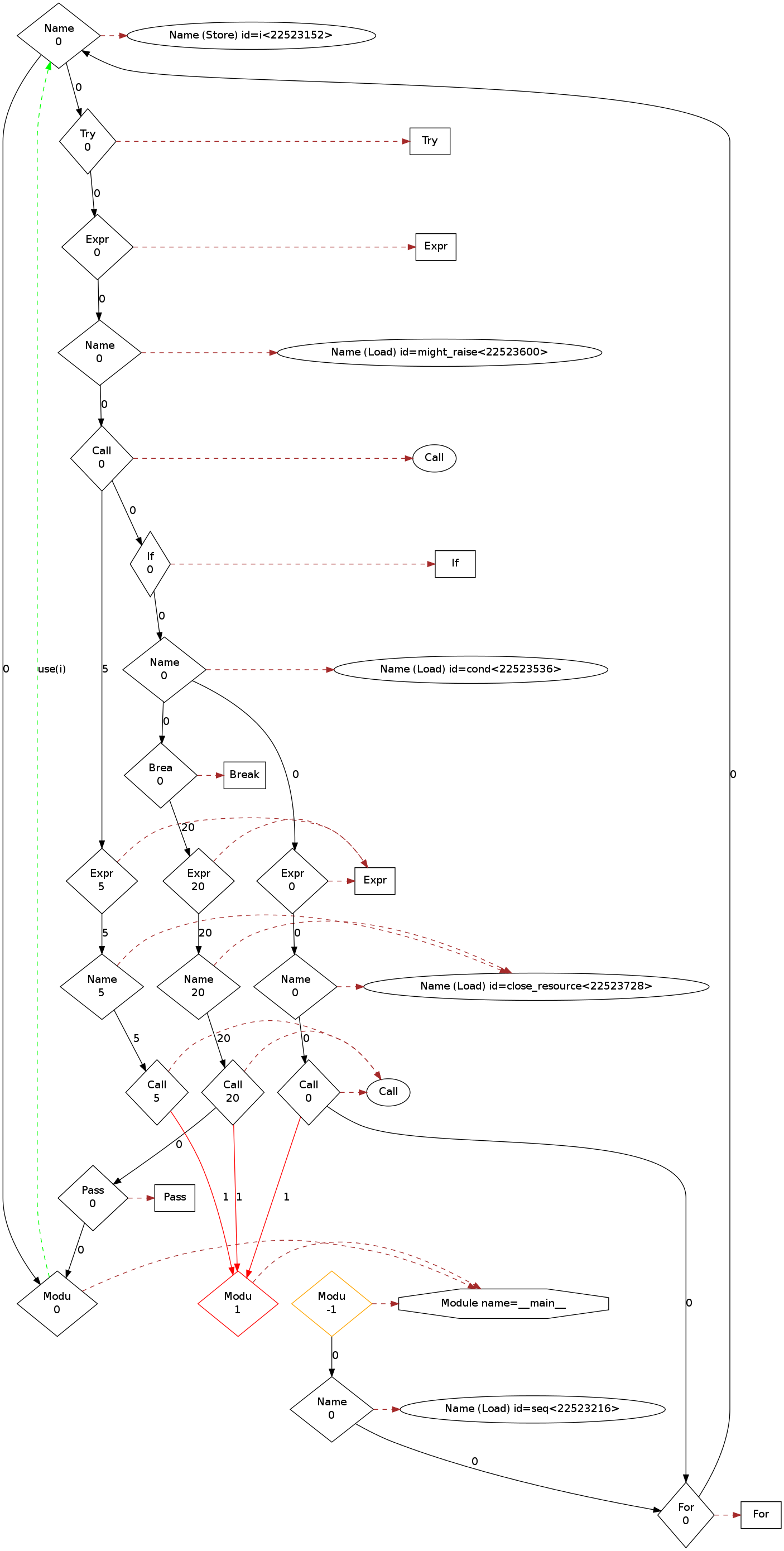# Analyzing control flow in Python¶

You can write CodeQL queries to explore the control-flow graph of a Python program, for example, to discover unreachable code or mutually exclusive blocks of code.

To analyze the control-flow graph of a `Scope` we can use the two CodeQL classes `ControlFlowNode` and `BasicBlock`. These classes allow you to ask such questions as “can you reach point A from point B?” or “Is it possible to reach point B without going through point A?”. To report results we use the class `AstNode`, which represents a syntactic element and corresponds to the source code - allowing the results of the query to be more easily understood. For more information, see Control-flow graph on Wikipedia.

## The `ControlFlowNode` class¶

The `ControlFlowNode` class represents nodes in the control flow graph. There is a one-to-many relation between AST nodes and control flow nodes. Each syntactic element, the `AstNode,` maps to zero, one, or many `ControlFlowNode` classes, but each `ControlFlowNode` maps to exactly one `AstNode`.

To show why this complex relation is required consider the following Python code:

```try:
might_raise()
if cond:
break
finally:
close_resource()
```

There are many paths through the above code. There are three different paths through the call to `close_resource();` one normal path, one path that breaks out of the loop, and one path where an exception is raised by `might_raise()`.

An annotated flow graph:The simplest use of the `ControlFlowNode` and `AstNode` classes is to find unreachable code. There is one `ControlFlowNode` per path through any `AstNode` and any `AstNode` that is unreachable has no paths flowing through it. Therefore, any `AstNode` without a corresponding `ControlFlowNode` is unreachable.

### Example finding unreachable AST nodes¶

```import python

from AstNode node
where not exists(node.getAFlowNode())
select node
```

Many codebases have some code that has no control flow node, and is therefore unreachable. However, since the `Module` class is also a subclass of the `AstNode` class, the query also finds any modules implemented in C or with no source code. Therefore, it is better to find all unreachable statements.

### Example finding unreachable statements¶

```import python

from Stmt s
where not exists(s.getAFlowNode())
select s
```

This query should give fewer results. You can also find unreachable code using the standard “Unreachable code” query. For more information, see Unreachable code.

## The `BasicBlock` class¶

The `BasicBlock` class represents a basic block of control flow nodes. The `BasicBlock` class is not that useful for writing queries directly, but is very useful for building complex analyses, such as data flow. The reason it is useful is that it shares many of the interesting properties of control flow nodes, such as, what can reach what, and what dominates what, but there are fewer basic blocks than control flow nodes - resulting in queries that are faster and use less memory. For more information, see Basic block and Dominator on Wikipedia.

### Example finding mutually exclusive basic blocks¶

Suppose we have the following Python code:

```if condition():
return 0
pass
```

Can we determine that it is impossible to reach both the `return 0` statement and the `pass` statement in a single execution of this code? For two basic blocks to be mutually exclusive it must be impossible to reach either of them from the other. We can write:

```import python

from BasicBlock b1, BasicBlock b2
where b1 != b2 and not b1.strictlyReaches(b2) and not b2.strictlyReaches(b1)
select b1, b2
```

However, by that definition, two basic blocks are mutually exclusive if they are in different scopes. To make the results more useful, we require that both basic blocks can be reached from the same function entry point:

```exists(Function shared, BasicBlock entry |
entry.contains(shared.getEntryNode()) and
entry.strictlyReaches(b1) and entry.strictlyReaches(b2)
)
```

Combining these conditions we get:

### Example finding mutually exclusive blocks within the same function¶

```import python

from BasicBlock b1, BasicBlock b2
where b1 != b2 and not b1.strictlyReaches(b2) and not b2.strictlyReaches(b1) and
exists(Function shared, BasicBlock entry |
entry.contains(shared.getEntryNode()) and
entry.strictlyReaches(b1) and entry.strictlyReaches(b2)
)
select b1, b2
```

This typically gives a very large number of results, because it is a common occurrence in normal control flow. It is, however, an example of the sort of control-flow analysis that is possible. Control-flow analyses such as this are an important aid to data flow analysis. For more information, see “Analyzing data flow in Python.”